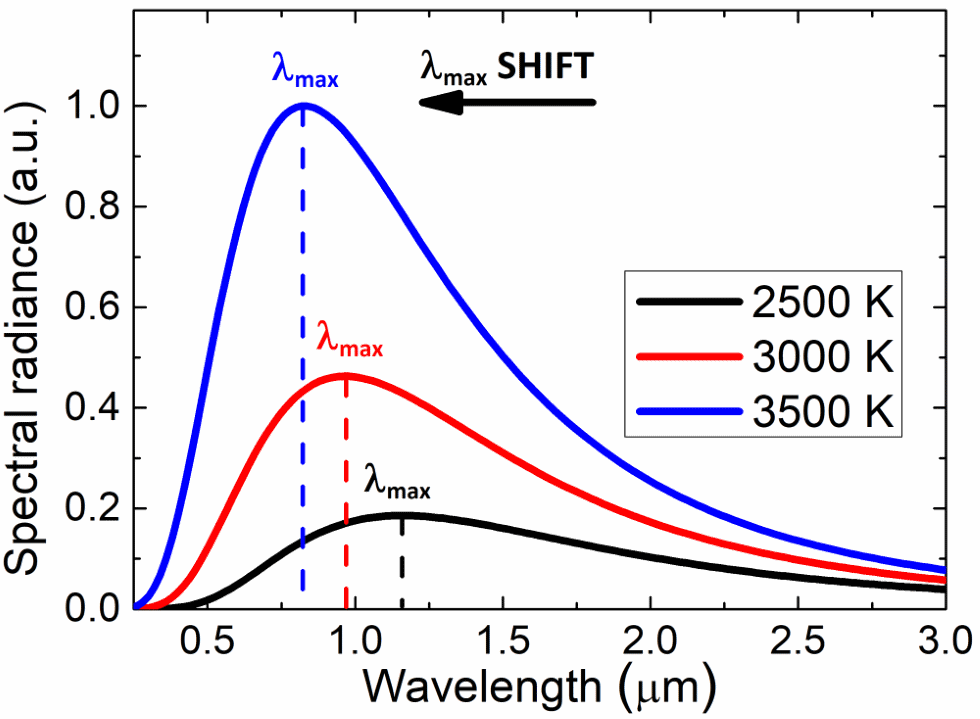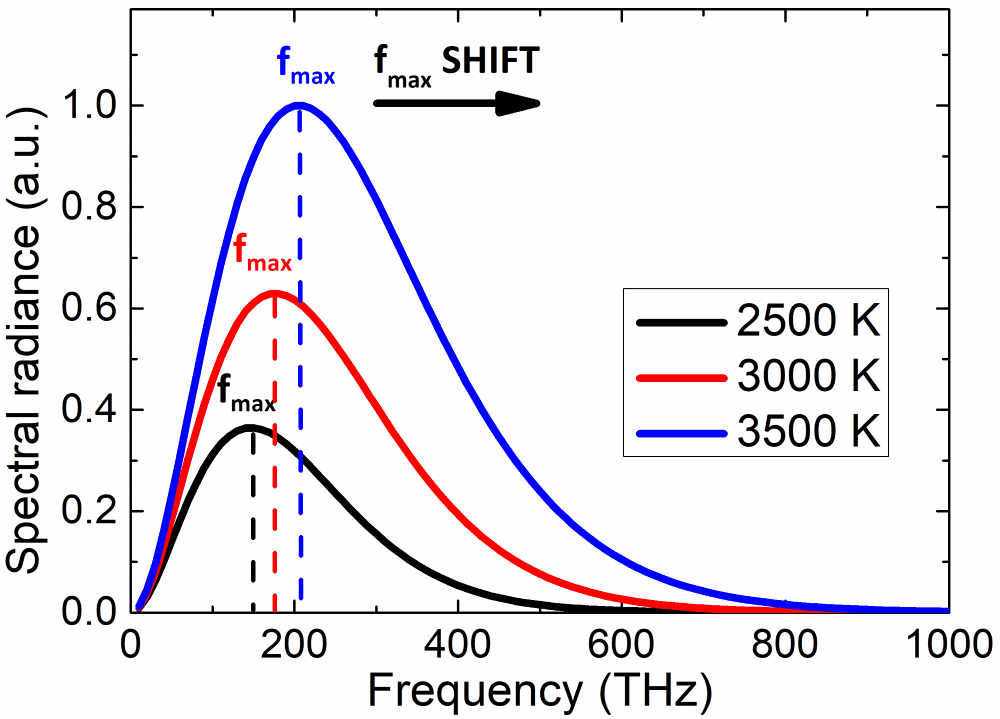# Wien's Law Calculator

Created by Wojciech Sas, PhD candidate
Reviewed by Bogna Szyk
Last updated: Dec 02, 2020

With this Wien's law calculator, you can easily estimate the temperature of an object, basing on the peak wavelength or frequency of its thermal emission spectrum. Read about Wien's displacement law, learn the Wien's law formula, and evaluate the temperature of Sun's surface, lava, or any hot body by yourself!

## Wien's displacement law

Wien's displacement law describes one of the relations between the emission spectrum of a black body and its temperature. It states that the higher the temperature, the lower the wavelength λmax for which the radiation curve reaches its maximum.
The shift to shorter wavelengths corresponds to photons of higher energies. In other words, λmax (peak wavelength) is inversely proportional to temperature.

The Stefan-Boltzmann law takes into account the total power radiated from a body at any temperature, and, together with the Wien's law, they originate from the Planck's distribution.

## Wien's law formula

The equation describing Wien's law is very simple:

λmax = b / T,

where:

• λmax is the aforementioned peak wavelength of light
• T is an absolute temperature of a black body
• b = 2.8977719 mm*K is the Wien's displacement constant

Although the relation between wavelength and frequency of electromagnetic waves is fairly simple (λ * f = c), we can't work out the peak frequency fmax by this analogy. The reason is that the spectral radiance is a sort of energy density function, so its shape and maximum depend on the argument (wavelength or frequency in our case). Knowing that the formula for the peak frequency is:

fmax = k * T,

where k = 5.8789232 * 10¹⁰ Hz/K is a numerical constant.

Wien's law formula can't be derived from classical physics. Numerous observations that confirm this law are among experiments (e.g., photoelectric effect), which contribute to the creation of quantum mechanics.## How to estimate the temperature of the Sun's surface? - example of use

Do you know how scientists can find the temperature of distant objects? Usually, they perform spectroscopic observation, fit Planck function to the measurement, and receive a parameter that is the temperature.

However, we can also get a nice estimation by applying the Wien's displacement law to the results. Let's try to calculate the temperature of the Sun's surface:

1. Find the peak wavelength of a solar spectrum. It's approximately λmax = 501.7 nm (or 5.017 * 10⁻⁷ m in the scientific notation).
2. Transform the Wien's law formula to obtain the temperature: T = b / λmax = 2.8977719 mm * K / 501.7 nm = 5776 K.

Although the black body is just an idealized model, the Wien's law is universal and can be a very accurate approximation for real objects. You can also work out the temperature of any body, like hot metal or lava, depending on the color of light it emits - use this Wien's law calculator and find out if the result surprises you or not!

Wojciech Sas, PhD candidate
Black body temperature
K
Peak wavelength
nm
Peak frequency
THz
People also viewed…

### BMR - Harris-Benedict equation

Harris-Benedict calculator uses one of the three most popular BMR formulas. Knowing your BMR (basal metabolic weight) may help you make important decisions about your diet and lifestyle.

### Grams to cups

The grams to cups calculator converts between cups and grams. You can choose between 20 different popular kitchen ingredients or directly type in the product density.

### Ideal gas density

Use this tool to calculate the density of an ideal gas using the ideal gas law.

### Thermal energy

With the thermal energy calculator, you can estimate the kinetic energy of molecules in an ideal gas.